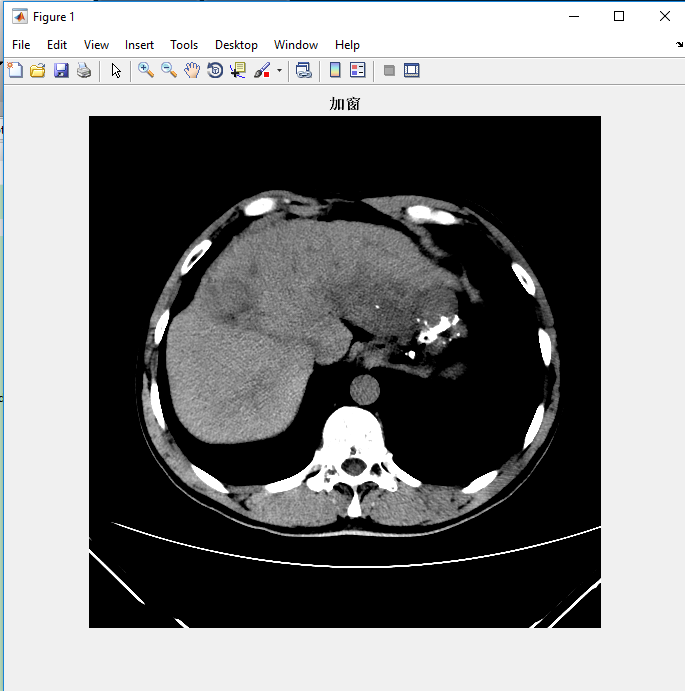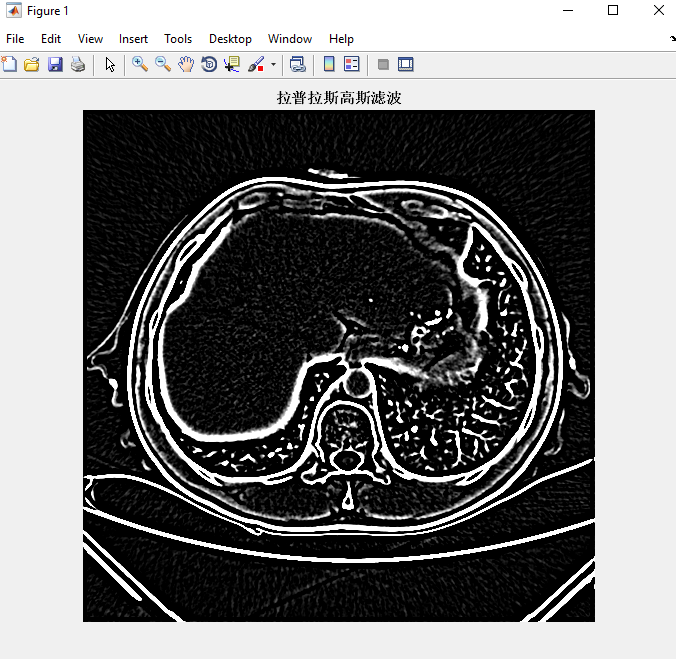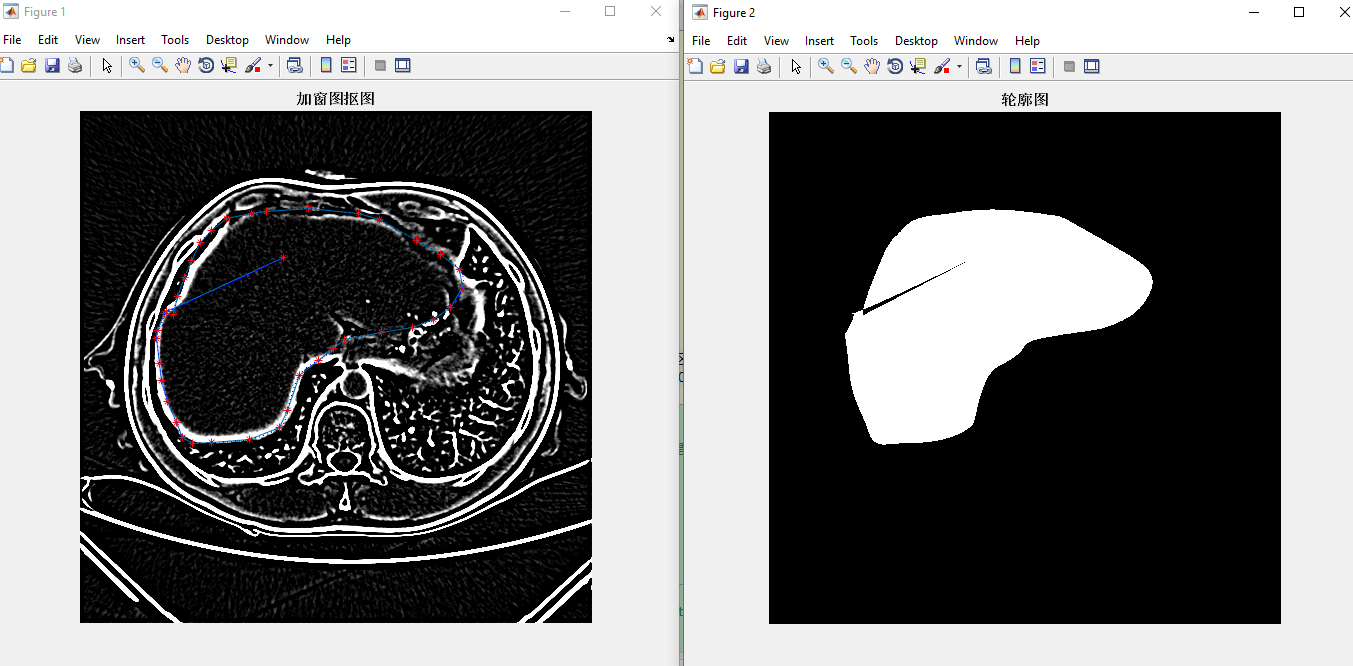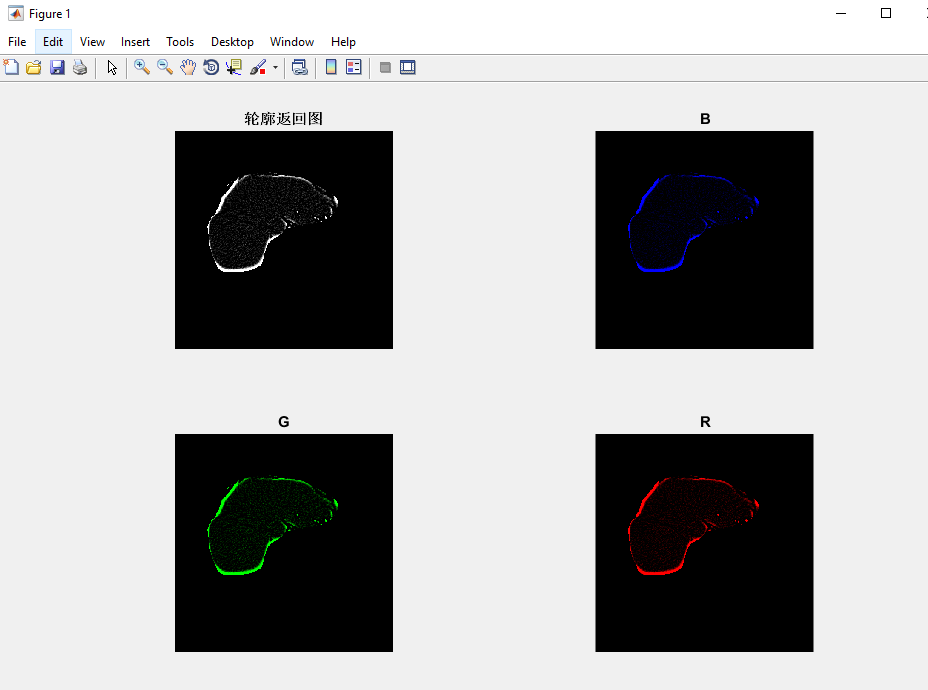# Matlab 高斯_拉普拉斯滤波器处理医学图像

## 前言：本程序是我去年实现论文算法时所做。主要功能为标记切割肝脏区域。时间有点久，很多细节已经模糊加上代码做了很多注释，因此在博客中不再详述。

NOTE: 程序分几大段功能模块，仔细阅读，对解决医学图像还是有一定的借鉴意义

.dcm数据，我放到了我的百度云盘，有兴趣的可以下载，实测一下代码。dcm数据连接

clc,clear
% figure
% imagesc(img_1);
% imshow(uint8(img_1));
Hu0=(int16(img_1)*1+(-1024));
%文档中的第二步，转为CT值的那个。
%% 窗宽窗位设置：c 窗位   w 窗宽  这个是按照你给的公式写的
Hu=double(Hu0);%此时有正负。转双精度的CT值。
gm=255;
c=60;
w=100;
Gv=0.*(Hu<c-w/2)+(gm/w)*(Hu+(w/2)-c).*(c-w/2<=Hu&Hu<=c+w/2)+gm.*(Hu>c+w/2);%Gv为显示灰度。
figure
imshow(uint8(Gv));
title('加窗')
%%   一次CTRL+T
%%  拉普拉斯高斯滤波 （有库函数）  %这个是整体区域。
img_gray=uint8(Gv);
hsize=10;%滤波模板大小。自己可以修改
sigma=0.4; %滤波系数sigma  自己修改会得到不同的效果图
h = fspecial('log', hsize, sigma); %构造拉普拉斯_高斯滤波器，'log'是这个滤波器的标志
img_filter0=double(imfilter(Hu0, h)); %调用matlab中的imfilter函数 ，进行滤波。其中img_gray为要过滤的图像，h为滤波器。
figure
img_filter=uint8(img_filter0);
imshow((img_filter));%显示图像
title('拉普拉斯高斯滤波')
%%
%% 自作ROI区域    利用ginput函数标出点，然后包围所标区域。%%对滤波变换后进行抠图
figure
imshow(img_filter);%%此处为img_filter 就是对滤波后抠图，此处为img_gray就是对为滤波的灰度图进行抠图。
title('加窗图抠图')
hold on
x=[];y=[];
n=0;
while(1)
[xtemp,ytemp,button]=ginput(1);
plot(xtemp,ytemp,'r*');
x=[x xtemp];
y=[y ytemp];
n=n+1;
%     text(xtemp+0.1,ytemp,int2str(n));
if(button==32)   %button=32表示  当你点点完了。你就按空格，退出点点的状态。
break
end
end
line(x,y);%x y连线
% hold off
t=1:n;
tt=1:0.1:n;
xx=spline(t,x,tt);  %因为手点 点数不够多，不够精确，需要插值  spline为插值函数
yy=spline(t,y,tt);
plot(xx,yy,'b:');
BW = roipoly(img_filter,xx,yy); %roipoly为抠图函数。以img_filiter为基础。xx yy为轮廓。包围的区域扣出来
figure, imshow(BW) %返回的是一个二值图像BW。就是一张黑白图。扣除的地方为1.其他地方均为0；
title('轮廓图')
%%  下面返回 被扣的地方的图像。 就是图上只有被扣除的东西
figure
img_last=BW.*(double(img_filter));%原理是这样：BW为 1 0的二值图，被扣的地方是兴趣区域都是1.这时
%与原图像进行.* ，除了为1的地方返回灰度值*1，其他地方都返回灰度值*0。
img_last1=uint8(img_last);%转为uint8格式。不然显示不了
img_last1(img_last1==0)=NaN;% 调整背景。
% figure
subplot(221)
imshow(img_last1)%显示最后的图像
title('轮廓返回图')
%%
%你需要的兴趣区域的数据如下： 。它的特点就是 兴趣区域为原图的CT值，非兴趣区域全是0；matlab图像是矩阵。所以必须是规则的。
%所以 要有0 来填充。
final_CT=BW.*(Hu);%%你要是要用 最后的抠图的CT值。这个就是。

%%论文中  有这么一句话：the mean gray-level intensity (m) and uniformity (u)
%%指的是平均灰度强度和均一性。实质求的是ROI区域的灰度值，和灰度值的均一性；
%% l是灰度值， p(l)是l灰度值出现的概率。
%% 先给图像加窗 转为灰度值Gv——>拉普拉斯高斯滤波——>抠图——>求抠图地方的灰度平均值m、均一性值n
%% 那个gui是演示用的。是在没滤波的基础上进行的抠图。没有关系。因为数据在这产生。
%%  img_last1 的最后数据。就是 抠出得图。周围用 0填充的。所以周围为黑色。

[p,q]=size(img_last1);
RGB=zeros(p,q);
img_B=cat(3,RGB,RGB,img_last1);%实质就是讲RGB  R=0，G=0，B=灰度值。下面类似。
subplot(222)
imshow(img_B);
title('B')
img_G=cat(3,RGB,img_last1,RGB);
subplot(223)
imshow(img_G);
title('G')
img_R=cat(3,img_last1,RGB,RGB);
subplot(224)
imshow(img_R);
title('R')

%%   ROI 区域均值求取。 img_mean;
img_sum=sum(img_last1(:));%求出所有元素总和。填充区域为0 。不影响。
N=numel(find(BW==1));%BW中有1的地方 就是有灰度的地方。所以BW中1 的多少，就是灰度的总数。
img_mean=img_sum/N;©️2019 CSDN 皮肤主题: 编程工作室 设计师: CSDN官方博客